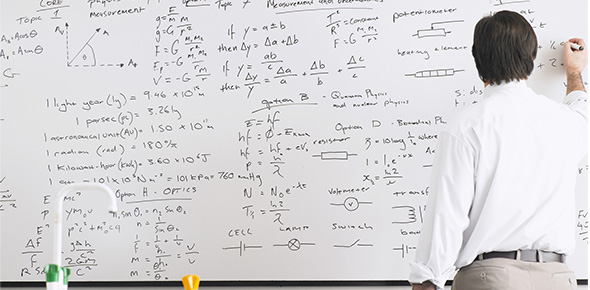# Equations Homework 4

5 QuestionsSettingsThis is practice for multi-step equations.

Related Topics
• 1.
Combine like terms. 4p + 14 = 11p
• A.

P = 2

• B.

P = 7

• C.

P = 14

• D.

P = 15

• 2.
Combine like terms. 3g – 6 = 4g – 7
• A.

G = 1

• B.

G = 7

• C.

G = 13

• D.

G = –13

• 3.
Combine like terms. 3(g – 2) = 7g – 18
• A.

G = 2

• B.

G = 3

• C.

G = –3

• D.

G = –2

• 4.
4x + 16 = 2x
• 5.
8x – 3 = 15 + 5x